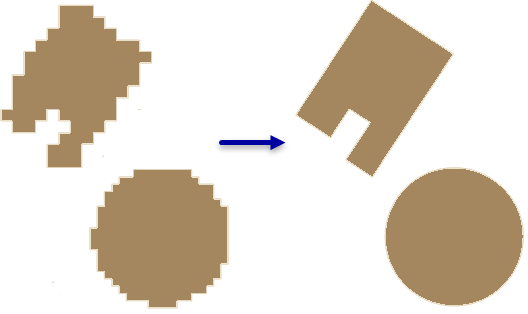# Regularize Building Footprint (3D Analyst)

## Summary

Normalizes the footprint of building polygons by eliminating undesirable artifacts in their geometry.

## Illustration## Usage

• This tool uses a polyline compression algorithm to correct distortions in building footprint polygons created through feature extraction workflows that may produce undesirable artifacts.

• If your building footprints contain circular structures, process those features first. A compactness ratio can be used to identify circular buildings. To calculate this value, do the following:

1. Add a field of type double.
2. Use the field calculator to compute the following formula:
``(4 * 3.14159265358979 * !shape.area!) / !shape.length! ** 2``
3. A perfect circle will have a value of 1; however, since the polygons typically processed with this tool have some irregularity, values closer to 1 are more likely to have a circular shape. Evaluate your results to identify the minimum value of a circular building and select values greater than or equal to this value prior to executing this tool with the Circle method.
• Consider using the Any Angle method if the building footprints represent geometries with edges that form a combination of angles that include, but are not limited to, 45° and 90° bends. Start by selecting a subset of features containing irregular edges and evaluate which diagonal penalty value yields the desired result. If the result does not adequately capture diagonal edges, consider modifying the tolerance value to constrain the regularization zone and incrementally lower the diagonal penalty. If the output contains undesirable edges with acute interior angles, iteratively run the tool while incrementally increasing the diagonal penalty until the desired output is attained, then use this value to process the entire dataset.

• When the Any Angles method is used on a computer with an NVIDIA graphics card that supports CUDA and has more than 2 GB of memory, the tool will use the GPU to perform its operation. You can modify this behavior in the Processor Type environment setting. If multiple GPUs are present, the desired GPU can be specified through the GPU ID environment setting.

• When the specified parameters cannot produce a regularized solution for a given input, the original feature is copied to the output. The value specified in the STATUS field will indicate whether the feature was regularized as follows:

• 0—Regularized feature
• 1—Original feature

## Syntax

`RegularizeBuildingFootprint(in_features, out_feature_class, method, tolerance, densification, precision, diagonal_penalty, min_radius, max_radius)`
 Parameter Explanation Data Type in_features The polygons that represent the building footprints to be regularized. Feature Layer out_feature_class The feature class that will be produced by this tool. Feature Class method The regularization method to be used in processing the input features. RIGHT_ANGLES —Constructs shapes comprised of 90° angles between adjoining edges.RIGHT_ANGLES_AND_DIAGONALS —Constructs shapes comprised of 45° and 90° angles between adjoining edges.ANY_ANGLE —Constructs shapes that form any angles between adjoining edges.CIRCLE —Constructs the best fitting circle around the input features. String tolerance The maximum distance that the regularized footprint can deviate from the boundary of its originating feature. The specified value will be based on the linear units of the input feature's coordinate system. Double densification The sampling interval that will be used to evaluate whether the regularized feature will be straight or bent. The densification must be equal to or less than the tolerance value. This parameter is only used with methods that support right angle identification. Double precision The precision of the spatial grid used in the regularization process. Valid values range from 0.05 to 0.25. Double diagonal_penalty When the RIGHT_ANGLES_AND_DIAGONALS method is used, this value dictates the likelihood of constructing right angles or diagonal edges between two adjoining segments. When the ANY_ANGLES method is used, this value dictates the likelihood of constructing diagonal edges that do not conform to the preferred edges determined by the tool's algorithm. Generally, the higher the value, the less likely a diagonal edge will be constructed. Double min_radius The smallest radius allowed for a regularized circle. A value of 0 implies there is no minimum size limit. This option is only available with the CIRCLE method. Double max_radius The largest radius allowed for a regularized circular. This option is only available with the CIRCLE method. Double

## Code sample

RegularizeBuildingFootprint example 1 (Python window)

The following sample demonstrates the use of this tool in the Python window.

``````arcpy.env.workspace = 'c:/data'
arcpy.ddd.RegularizeBuildingFootprint('rough_footprints.shp',
'regularized_footprints.shp',
RegularizeBuildingFootprint example 2 (stand-alone script)

The following sample demonstrates the use of this tool in a stand-alone Python script.

``````'''****************************************************************************
Name: Classify Lidar & Extract Building Footprints
Description: Extract footprint from lidar points classified as buildings,
regularize its geometry, and calculate the building height.

****************************************************************************'''
import arcpy

lasd = arcpy.GetParameterAsText(0)
dem = arcpy.GetParameterAsText(1)
footprint = arcpy.GetParameterAsText(2)

try:
desc = arcpy.Describe(lasd)
if desc.spatialReference.linearUnitName in ['Foot_US', 'Foot']:
unit = 'Feet'
else:
unit = 'Meters'
ptSpacing = desc.pointSpacing * 2.25
sampling = '{0} {1}'.format(ptSpacing, unit)
# Classify overlap points
arcpy.ddd.ClassifyLASOverlap(lasd, sampling)
# Classify ground points
arcpy.ddd.ClassifyLasGround(lasd)
# Filter for ground points
arcpy.management.MakeLasDatasetLayer(lasd, 'ground', class_code=)
# Generate DEM
arcpy.conversion.LasDatasetToRaster('ground', dem, 'ELEVATION',
'BINNING NEAREST NATURAL_NEIGHBOR',
sampling_type='CELLSIZE',
sampling_value=desc.pointSpacing)
# Classify noise points
arcpy.ddd.ClassifyLasNoise(lasd, method='ISOLATION', edit_las='CLASSIFY',
withheld='WITHHELD', ground=dem,
low_z='-2 feet', high_z='300 feet',
max_neighbors=ptSpacing, step_width=ptSpacing,
step_height='10 feet')
# Classify buildings
arcpy.ddd.ClassifyLasBuilding(lasd, '7.5 feet', '80 Square Feet')
#Classify vegetation
arcpy.ddd.ClassifyLasByHeight(lasd, 'GROUND', [8, 20, 55],
compute_stats='COMPUTE_STATS')
# Filter LAS dataset for building points
lasd_layer = 'building points'
arcpy.management.MakeLasDatasetLayer(lasd, lasd_layer, class_code=)
# Export raster from lidar using only building points
temp_raster = 'in_memory/bldg_raster'
arcpy.management.LasPointStatsAsRaster(lasd_layer, temp_raster,
'PREDOMINANT_CLASS', 'CELLSIZE', 2.5)
# Convert building raster to polygon
temp_footprint = 'in_memory/footprint'
arcpy.conversion.RasterToPolygon(temp_raster, temp_footprint)
# Regularize building footprints
arcpy.ddd.RegularizeBuildingFootprint(temp_footprint, footprint,
method='RIGHT_ANGLES')

except arcpy.ExecuteError:
print(arcpy.GetMessages())``````

## Licensing information

• Basic: Requires 3D Analyst
• Standard: Requires 3D Analyst